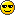Table after WellingTable after Welling

Attendance  1585

Oh we were all trying so hard to be optimistic, weren't webut the only person to come (eerily) close was Ashley.
Well done, AshleyYou only narrowly missed out on BIG pointsbut it has meant that you have climbed the table to 3rdMa Gibbons   55 + 0 = 55
BenE    50 + 0 = 50
Ashley  5 + 25 = 30
Bristol Mike   25 + 0 = 25
LB    25 + 0 = 25
Midsomer-Chris  25 + 0 = 25
Claire K  20 + 0 = 20
Rabbit  20 + 0 = 20
Comrade Powell  15 + 0 = 15
Kermit   15 + 0 = 15
Midsomer-Steve    10 + 0 = 10
Beau Nash  5 + 0 = 5
Luton Roman   5 + 0 = 5
Azlimey   0 + 0 = 0
Maurice Ashman 0
PhilB1973   0 + 0 = 0
Sean   0 + 0 = 0
2Weirdtown   0 + 0 = 0Midsomer-chris

Posts : 841
Join date : 2014-02-21
Age : 60Permissions in this forum:
You cannot reply to topics in this forum